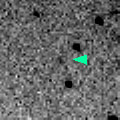# \$B%j%K%"WB@1(B

C/1998 W3 ( LINEAR )###\$B%W%m%U%#!<%k(B

 \$BH/8+F|(B 1998\$BG/(B11\$B7n(B25\$BF|(B \$BH/8+8wEY(B 18.4\$BEy(B \$BH/8+ Lincoln Laboratory Near-Earth Asteroid Research project

###\$B###\$B50F;MWAG(B

```   The following improved orbital elements by Kenji Muraoka, are
from 141 observations 1998 Nov. 25 to 1999 Sept. 13, perturbations
by 9 Planets, Moon and 5 minor planets were taken into account.
The mean residual is +/- 0.54 arc seconds.

Epoch  =  1998 Sept. 24.0  TT      JDT = 2451080.5
T  =  1998 Oct.   6.73963      +/- 0.00919 (m.e.) TT
Peri. =    6.88974                +/- 0.00101
Node  =  123.91860                +/- 0.00076   (2000.0)
Incl. =  129.19152                +/- 0.00078
q  =    4.9145993              +/- 0.0000121 AU
e  =    1.0012517              +/- 0.0000276
1/a  =   -0.0002547              +/- 0.0000056 1/AU
orig. 1/a  =   +0.000248
fut.  1/a  =   +0.000065
```

###\$B@1?^(B###\$B8wEYJQ2=(B

```        m1 = 6.5 + 5 log\$B&\$(B + 10.0 log r
```##### \$B50F;MWAG\$OB<2,7r<#;a\$N7W;;\$K\$h\$k\$b\$N\$G\$9!#(B \$B@1?^\$O(B StellaNavigator Ver.2.0 for Windows (\$B%"%9%H%m%"!<%D(B \$BJTCx(B / \$B%"%9%-!<=PHG6I4)(B) \$B\$G:n@.\$7\$?\$b\$N\$G\$9!#(B \$B8wEY%0%i%U\$O(BComet for Windows\$B\$G:n@.\$7\$?\$b\$N\$G\$9!#(B